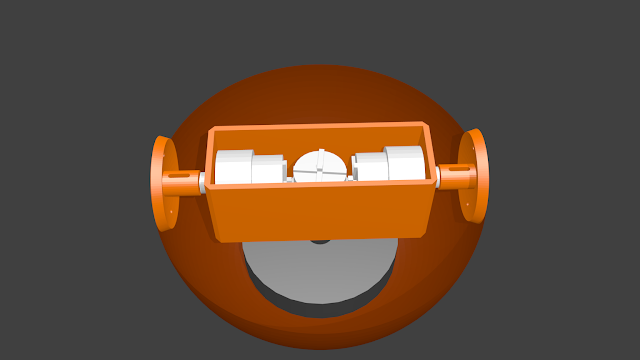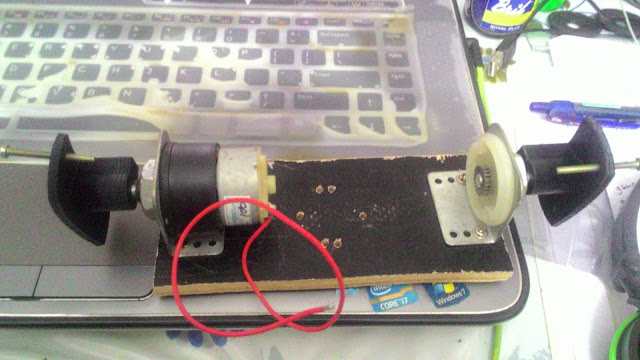## Saturday, 27 February 2016

### My First Build of BB-8

Being a robotics person, the part of Star Wars: The Force Awakens that gave me the most delight was BB-8! The cute little droid who rolls along on a sphere. The moment I saw it on screen I knew that I had to build it! This blogpost documents my entire build process.

I decided that the first thing I should do is come up with a good control mechanism. The first thing I thought of was a pendulum hanging from a diametric bar inside the sphere. The pendulum would be free hanging and an actuator would apply torque on it and make the sphere roll. This seemed a simple enough system to work with. So I started the process of simulating the robot and coming up with a good control system.

### The Mathy Stuff

The easiest way to model a sort of complex system like this is to use Lagrangian mechanics. Write down the difference between the kinetic and potential energies of the system, apply the Euler-Lagrange equations to get the equations of motion and plug the equations into a differential equation with initial conditions. This general procedure can be used to simulate a wide range of mechanical systems. It's an essential part of any roboticist's toolkit.

In the case of the BB-8 sphere pendulum system the equations of motion come out as:

$\frac{5}{3} m_b \ddot{x}(t)+m_p \left(l \ddot{\theta}(t) \cos (\theta (t))-l \dot{\theta}(t)^2 \sin (\theta (t))+\ddot{x}(t)\right)=\frac{u(t)}{r}$
$l m_p \left(g \sin (\theta (t))+l \ddot{\theta}(t)+\cos (\theta (t)) \ddot{x}(t)\right)=-u(t)$

Here $m_b$ is the mass of the ball, $m_p$ is the mass of the pendulum, $l$ the length of the pendulum rod and $r$ the radius of the sphere. The state of the robot is represented by the state vector $\left[ \begin{array}{c} x \\ \theta \\ \dot{x} \\ \dot{\theta} \end{array} \right]$ where $x$ is the distance the sphere has rolled and $\theta$ is the angle the pendulum makes with the vertical.

When the motor applies a torque on the pendulum, it also applies an equal and opposite torque on the shaft on the spherical body. This is the control torque we're going to use to make BB-8 roll back and forth. For the mass of the pendulum I am using another motor with a weighted disk. This motor can react against the weighted disk to make the robot turn left and right. These two mechanisms are enough to make BB-8 go anywhere! Since this robot is an open chain manipulator, the equations of motion can be written in the manipulator equation form $H(q)\ddot{q} + C(q,\dot{q})\dot{q} + G(q) = Bu$.

$\left[\begin{array}{cc}\frac{5}{3}m_b + m_p & m_pl\cos\theta \\ m_pl\cos\theta & m_pl^2\end{array}\right] \left[\begin{array}{c}\ddot{x} \\ \ddot{\theta}\end{array}\right] + \left[\begin{array}{cc}0 & -m_pl\dot{\theta}\sin\theta \\ 0 & 0 \end{array}\right] \left[\begin{array}{c}\dot{x} \\ \dot{\theta}\end{array}\right] + \left[\begin{array}{c}0 \\ m_pgl\sin\theta\end{array}\right] = \left[\begin{array}{c} \frac{1}{r} \\ -1 \end{array}\right] u$

This is what the system looks like without any motors.

We can easily write the manipulator equation to the standard form ( $\dot{x} = f(x,u)$ ), linearize it around any equilibrium point to get a linear state space model and implement a linear controller. I'm so glad that I had Mathematica to help me with the modeling. It made things very simple and I could get a simple LQR for controlling the sphere working without too much hassle. Here's what the motion of the sphere looks like with the controller.

### The Body

Now it's time to build! First I was stuck on what to make the sphere out of. I didn't want the robot to be too small and at first, I couldn't think of any place to find a sphere. So i started designing a spherical shell in Blender. I had to do it in parts because of size constraints on the 3D printer. This was very time consuming. And then it struck me! I could use on of these! :D

I bought a 20 cm diameter globe off amazon. The sphere is made out of ABS plastic which is really nice. I used a hacksaw to cut the sphere in half. The next challenge is to mount the motors. I tried reusing empty potato chip cans but most of them didn't have the dimensions that I wanted. So in the end I decided to 3D print a motor housing and some parts to couple the sphere to the motors. I used Blender (Open Source! Yay! :D) to design the parts.Initial Render of what the internals were going to look like.
However, it turned out that 3D printing parts is a bit time consuming. Also, the motor couplings were difficult to print without using a lot of support material. After a few iterations of redesigning and checking, the final motor coupling was printed. I made a decent looking motor housing out of some wood and metal L-shaped motor mounts.The motor assembly

### The Electronics

An Arduino Nano with an Atmega328 is the brain of the robot. A GY521 accelerometer/gyro board helps me measure the angle of the motor assembly with respect to the ground and a rotary encoder coupled to the dead axle helps me measure the speed and position of the ball. A HC05 Bluetooth module communicates with an Android app that I made using MIT App Inventor so that I can control the robot.

Putting it all together lead to this huge mess of wires.

After multiple attempts at rewiring, I got to this slightly less messy (but still quite messy) state.The battery is also included in this one.
Despite the messiness, it actually works! :D I made it roll around for a bit.

Since this was my first build, I decided that it should more of a proof of concept than a robust build. So I built it very fast. Now  that I know that it works, I can spend some time designing a nice PCB to make the wiring neater and more compact. I also need to fine tune the control loop so that the whole thing moves smoothly. No point in tuning it on this since I'll be rebuilding a part of it anyway.

Watch out for the next post about a more robust build!